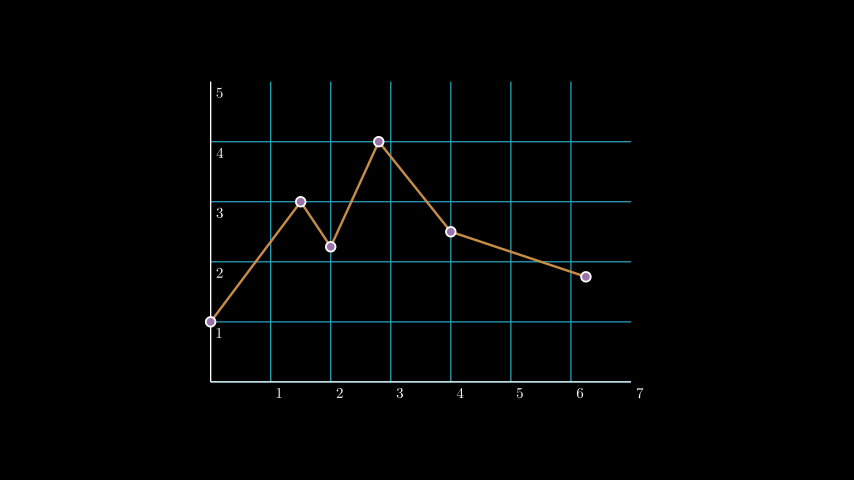# manim.mobject.coordinate_systems.Axes¶

class `Axes`(x_range=None, y_range=None, x_length=12, y_length=6, axis_config=None, x_axis_config=None, y_axis_config=None, tips=True, **kwargs)[source]

Creates a set of axes.

Parameters
• x_range (Optional[Sequence[float]]) – The `[x_min, x_max, x_step]` values of the x-axis.

• y_range (Optional[Sequence[float]]) – The `[y_min, y_max, y_step]` values of the y-axis.

• x_length (Optional[float]) – The length of the x-axis.

• y_length (Optional[float]) – The length of the y-axis.

• axis_config (Optional[dict]) – Arguments to be passed to `NumberLine` that influences both axes.

• x_axis_config (Optional[dict]) – Arguments to be passed to `NumberLine` that influence the x-axis.

• y_axis_config (Optional[dict]) – Arguments to be passed to `NumberLine` that influence the y-axis.

• tips (bool) – Whether or not to include the tips on both axes.

• kwargs (Any) – Additional arguments to be passed to `CoordinateSystem` and `VGroup`.

Methods

 `coords_to_point` Transforms the vector formed from `coords` formed by the `Axes` into the corresponding vector with respect to the default basis. `create_axis` Creates an axis and dynamically adjusts its position depending on where 0 is located on the line. `get_axes` Gets the axes. `get_line_graph` Draws a line graph. `origin_shift` Determines how to shift graph mobjects to compensate when 0 is not on the axis. `point_to_coords` Transforms the coordinates of the point which are with respect to `manim`’s default basis into the coordinates of that point with respect to the basis defined by `Axes`. `update_default_configs`

Attributes

 `animate` Used to animate the application of a method. `animation_overrides` `depth` The depth of the mobject. `height` The height of the mobject. `width` The width of the mobject.
`coords_to_point`(*coords)[source]

Transforms the vector formed from `coords` formed by the `Axes` into the corresponding vector with respect to the default basis.

Returns

A point that results from a change of basis from the coordinate system defined by the `Axes` to that of `manim`’s default coordinate system

Return type

np.ndarray

Parameters

coords (Sequence[float]) –

`create_axis`(range_terms, axis_config, length)[source]

Creates an axis and dynamically adjusts its position depending on where 0 is located on the line.

Parameters
• range_terms (Sequence[float]) – The range of the the axis : (x_min, x_max, x_step).

• axis_config (dict) – Additional parameters that are passed to `NumberLine`.

• length (float) – The length of the axis.

Returns

Returns a number line with the provided x and y axis range.

Return type

`NumberLine`

`get_axes`()[source]

Gets the axes.

Returns

A pair of axes.

Return type

`VGroup`

`get_line_graph`(x_values, y_values, z_values=None, line_color='#FFFF00', add_vertex_dots=True, vertex_dot_radius=0.08, vertex_dot_style=None, **kwargs)[source]

Draws a line graph.

The graph connects the vertices formed from zipping `x_values`, `y_values` and `z_values`. Also adds `Dots` at the vertices if `add_vertex_dots` is set to `True`.

Parameters
• x_values (Iterable[float]) – Iterable of values along the x-axis.

• y_values (Iterable[float]) – Iterable of values along the y-axis.

• z_values (Optional[Iterable[float]]) – Iterable of values (zeros if z_values is None) along the z-axis.

• line_color (colour.Color) – Color for the line graph.

• add_vertex_dots (bool) – Whether or not to add `Dot` at each vertex.

• vertex_dot_radius (float) – Radius for the `Dot` at each vertex.

• vertex_dot_style (Optional[dict]) – Style arguments to be passed into `Dot` at each vertex.

• kwargs – Additional arguments to be passed into `VMobject`.

Return type

manim.mobject.types.vectorized_mobject.VDict

Examples

Example: LineGraphExample```from manim import *

class LineGraphExample(Scene):
def construct(self):
plane = NumberPlane(
x_range = (0, 7),
y_range = (0, 5),
x_length = 7,
axis_config={"include_numbers": True},
)
plane.center()
line_graph = plane.get_line_graph(
x_values = [0, 1.5, 2, 2.8, 4, 6.25],
y_values = [1, 3, 2.25, 4, 2.5, 1.75],
line_color=GOLD_E,
vertex_dot_style=dict(stroke_width=3,  fill_color=PURPLE),
stroke_width = 4,
)
```
static `origin_shift`(axis_range)[source]

Determines how to shift graph mobjects to compensate when 0 is not on the axis.

Parameters

axis_range (Sequence[float]) – The range of the axis : `(x_min, x_max, x_step)`.

Return type

float

`point_to_coords`(point)[source]

Transforms the coordinates of the point which are with respect to `manim`’s default basis into the coordinates of that point with respect to the basis defined by `Axes`.

Parameters

point (float) – The point whose coordinates will be found.

Returns

Coordinates of the point with respect to `Axes`’s basis

Return type

Tuple